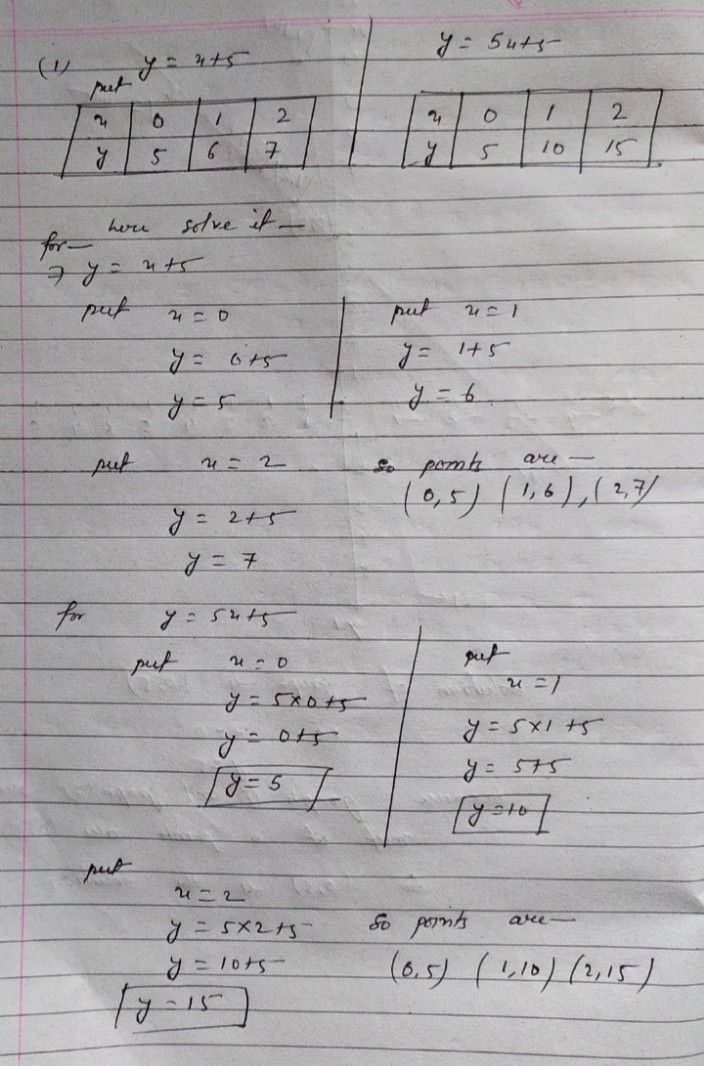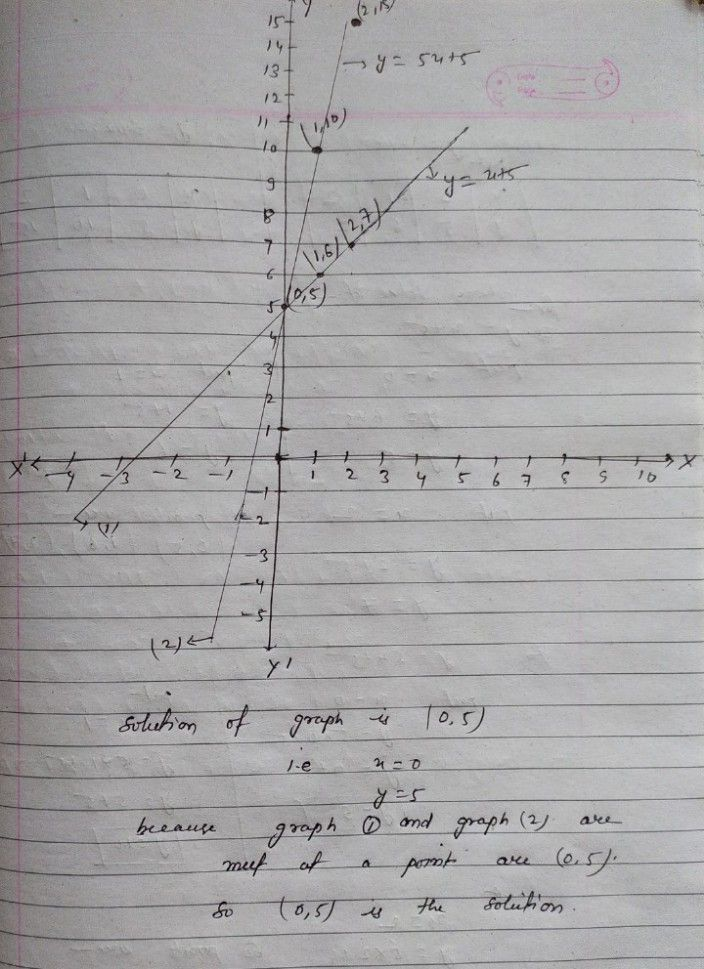Symbol
ProblemLearning Task $3$ Graph the system of equations $1$ $y=x+5$ and $y=5x+5$ $2$ $x-3y=-12$ and $4x+6y=-12$ $3$ $x+2y=-5$ and $-2x+y=3$ $4$ $y=3x-2$ and $y=-x-6$ $5$ $2x-3y=-2$ and $4x+y=24$
7th-9th grade
Algebra
Search count: 117
SolutionQanda teacher - missPreetiIf you have any doubts please do let me know dear. if you are satisfied with my answer please do LIKE and give a 5 STAR RATING and coins as THANK U GIFT..! ALL THE BEST..!!!If you have more questions then please ask through preffered match from my profileStudent
thank you so muchQanda teacher - missPreeti
To evaluate:- 1. Click on top right corner evaluate button. 2. Select 5 star 3. Click on gift coins + three times. 4. Click on DONE button on top right corner.